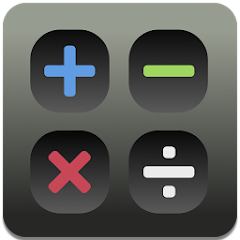# Desktop Calculator C

10K+Everyone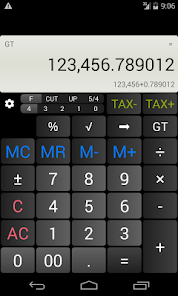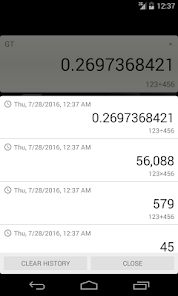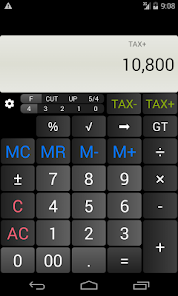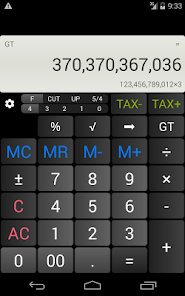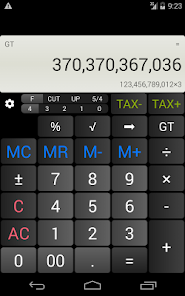Features:
Independent Memory, Square Root, Change Sign (+/-), Tax Calculation, Percent Calculation, Constant Calculation, Grand Total, Slide Switch(Rounding), Calculation result history

- TAX calculation
(1) How much is Price-plus-Tax of 1000?
Input 1000 [TAX+].
(2) How much is the Price-less-Tax of 1080?
Input 1080 [TAX-].
* You can check Tax rate by pressing [TAX+]

- Slide Switch(Rounding)
F: Floating Decimal
CUT: Values are cut off to the number of decimal places specified by the current Decimal Place Selector setting
UP: Values are rounded up to the number of decimal places specified by the current Decimal Place Selector setting
5/4: Values are rounded off (0,1,2,3,4 are cut off; 5,6,7,8,9 are rounded up) to the number of decimal places specified by the current Decimal Place Selector setting
4,3,2,1,0: Specifies the number of decimal places as 4,3,2,1,0

- Percent Calculation
Adding 10%: 1000 [×] 10 [%] [+]
Subtract 10%: 1000 [×] 10 [%] [-]

1000+10%: This is a markup on price procedure. The formula is as follows:
Markup%=(Markup Price-Cost)/(Markup Price)

- Grand Total
Whenever the “=” Key has been pressed, the result will be stored into the Grand Total Memory. The “GT” Key is to recall the Grand Total Memory. It’s function is similar to the “MR” Key, both of them are used for recalling the Memory Content.

- Constant Calculation
Press "+", "-", "×", "÷" twice to register a constant. The "K" indicator is displayed to indicate that Constant Calculation is in effect. This will reverse the order of the input values, for example, if you input 4--3, the result of the constant calculation will be 3-4.

Updated on
Sep 30, 2021

## Data safety